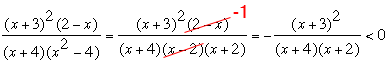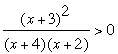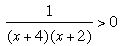SEARCH HOMEMath Central Quandaries & QueriesQuestion from ed, a student: solve the inequality (x+3)^2 (2-x) ___________ < 0 (x+4) (x^2 - 4)Hi Ed,

What I notice first is the difference of squares x2 - 4 = (x - 2)(x + 2) in the denominator and (2 - x) in the numerator so I am going to have some cancellation.You know that this cancellation is invalid if x - 2 = 0, that is x = 2 so when you are done you nee to check that x can't be 2.

Now multiple each side by -1 which changes the direction of the inequality and leaves us withNow I see that (x + 3)2 can't be negative so I can divide both sides by (x + 3)2 to obtainAgain this step is invalid if x = -3 and in fact x = -3 does not satisfy the original inequality so you need to insure when you report the final result that x = -3 is not given as a solution.

If the inequality above is true then either both (x + 4) and (x + 2) are positive or they are both negative. Can you complete the problem now?

If you need more assistance write back,
PennyMath Central is supported by the University of Regina and The Pacific Institute for the Mathematical Sciences.